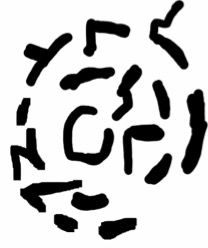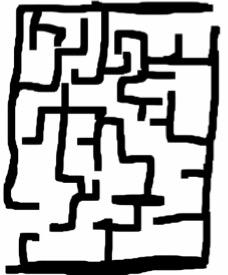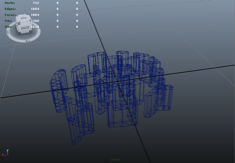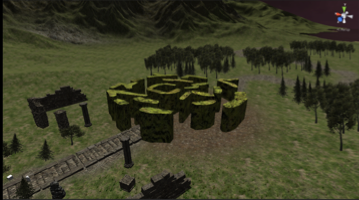# Metoria Game Design: Designing the MazeIn this section I will explain how I created the maze. In order to design the maze, research was done in order to know what the maze structure looks like. Based on my research I discovered in order to design a successful maze, the user must be able to enter and navigate through the other side. The structure of the maze will determine the complexity of the navigation.

After researching maze structures, I created some concepts of various maze structures as shown below in figure 1.Figure 1: Concept design drawings of mazes

The next step is to create the maze. In order to do this, I used using maya tools and the sketched maze map as an outline to create a mesh of my maze in Maya Autodesk. The result is shown below in figure 2.Figure 2: Maze model: Wireframe

Once the model is created, I then applied a texture to the model. The result is shown below in figure 3.Figure 3: Maze model textured

Once the model is textured, I then exported the model into Unity as an asset. The model asset is then placed into the game and resized and below is the end result in figure 4.Figure 4: Maze model in the environment